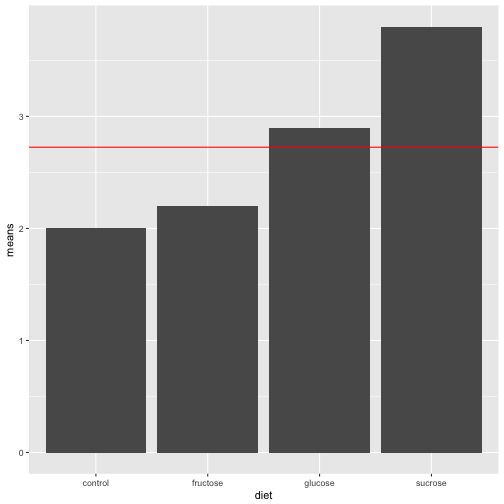# Formal ANOVA

Prof Randi Garcia
February 19, 2018

1. How do we proceed to calculate F-ratios if we only have a set of sums of squares (SS) and degrees of freedom (df)?
2. Exaplin why chance error is part of the MS calculation for the factor of interest. How does this relate to how the F-ratio is constructed?
3. If you have time, write any questions you have from the reading, or ideas that you feel fuzzy on.

### Announcements

• HW 3 due tonight
• Project proposal due in 1 week
• HW 4, due 1 week from Wed
• Start it now!
• Some CH 4 questions

### Agenda

• Assumptions
• Decomposition
• Analysis of variance (ANOVA)
• SS, MS, df, F

### C. Constant effects

We assume every observation in a similar condition is affected exactly the same. (Gets the same true score).

animals_sim <- animals %>%
mutate(benchmark = mean(calm)) %>%
group_by(animal) %>%
mutate(animal_mean = mean(calm),
aminal_effect = animal_mean - benchmark)


We add the effects as we go down the assembly line.

The interaction effect captures the possibility that conditions have non-additive effects, but it is also added to everything else.

calm_sim = benchmark
+ aminal_effect
+ cue_effect
+ interaction_effect
+ student_effect


### S. Same standard deviations

The piece of code for adding error is not dependent on which condition the observation is in.

 + rnorm(64, 0, 0.65)


### I. Independent residuals

Takes 64 independent draws from a normal distribution.

 + rnorm(64, 0, 0.65)


### N. Normally distributed residuals

It's rnorm(), and not rbinom() or rpois()

 + rnorm(64, 0, 0.65)


### Z. Zero mean residuals

The second argument is the mean.

 + rnorm(64, 0, 0.65)


### Leafhopper survival

It is reasonable to assume that the structure of a sugar molecule has something to do with its food value. An experiment was conducted to compare the effects of four sugar diets on the survival of leafhoppers. The four diets were glucose and fructose (6-carbon atoms), sucrose (12-carbon), and a control (2% agar). The experimenter prepared two dishes with each diet, divided the leafhoppers into eight groups of equal size, and then randomly assigned them to dishes. Then she counted the number of days until half the insects had died.

### Decomposing the data

control sucrose glucose fructose
2.3 3.6 3.0 2.1
1.7 4.0 2.8 2.3
• Draw the factor diagram, including the benchmark and residuals.

### Decomposing the data• We need to start thinking about if those differences in treatment means are real, or could possibly be due to chance error.
• To your factor diagram, let's add in the benchmark, the effects for diet, and the residuals
X. control sucrose glucose fructose
2.3 3.6 3.0 2.1
1.7 4.0 2.8 2.3
means 2.0 2.2 2.9 3.8

### Analysis of Variance ANOVA

Formal ANOVA starts with the simple idea that we can compare our estimate of treatment effect variability to our estimate of chance error variability to measure how large our treatment effect is.

Variability in treatment effects = True Effect Differences + Error

Variability in residuals = Error

• If our null hypothesis is, $${H}_{0}$$: True Effect Differences $$=0$$, then what would we expect the following ratio to equal?

Variability in treatment effects/Variability in residuals

### Sum of Squares (SS)

ANOVA measures variability in treatment effects with the sum of squares (SS) divided by the number of units of unique information (df). For the BF design,

${SS}_{Treatments} = n\sum_{i=1}^{a}(\bar{y}_{i.}-\bar{y}_{..})^{2}$

${SS}_{E} = \sum_{i=1}^{a}\sum_{j=1}^{n}({y}_{ij}-\bar{y}_{i.})^{2}$

${SS}_{Total} = {SS}_{Treatments} + {SS}_{E}$

where $$n$$ is the group size, and $$a$$ is the number of treatments.

### Degrees of Freedom (df)

The df for a table equals the number of free numbers, the number of slots in the table you can fill in before the pattern of repetitions and adding to zero tell you what the remaining numbers have to be.

${df}_{Treatments}=a-1$

${df}_{E}=N-a$

### Mean Squares (MS)

The ultimate statistic we want to calculate is Variability in treatment effects/Variability in residuals.

Variability in treatment effects: ${MS}_{Treatments}=\frac{{SS}_{Treatments}}{{df}_{Treatments}}$

Variability in residuals ${MS}_{E}=\frac{{SS}_{E}}{{df}_{E}}$

### F-ratios and the F-distribution

The ratio of these two MS's is called the F ratio. The following quantity is our test statistic for the null hypothesis that there are no treatment effects.

$F = \frac{{MS}_{Treatments}}{{MS}_{E}}$

If the null hypothesis is true, then F is a random variable $$\sim F({df}_{Treatments}, {df}_{E})$$. The F-distribution.

qplot(x = rf(500, 3, 4), geom = "density")


We can find the p-value for our F calculation with the following code

pf(17.67, 3, 4, lower.tail = FALSE)


### Inside-outside Factors

• We cannot always use the same formula for the treatment effects. It depends on inside and outside factors
• Estimated effect for a factor = Average for the factor - sum of estimated effects for all outside factors. That is,

$Effect = Average - Partial Fit$

• One factor is inside another if each group of the first (inside) fits completely inside some group of the second (outside) factor.

### Inside-outside Factors

student animal cute scary
2 cat 5 1
5 cat 5 5
1 dog 5 1
3 dog 4 2
• Draw the factor diagram as a hierarchy of inside and outside factors

### Start HW 4

• Ch4: B1-3, C3, D1, RE CH 3: 3-4 (data in fig 3.21), 11-13, 17-19